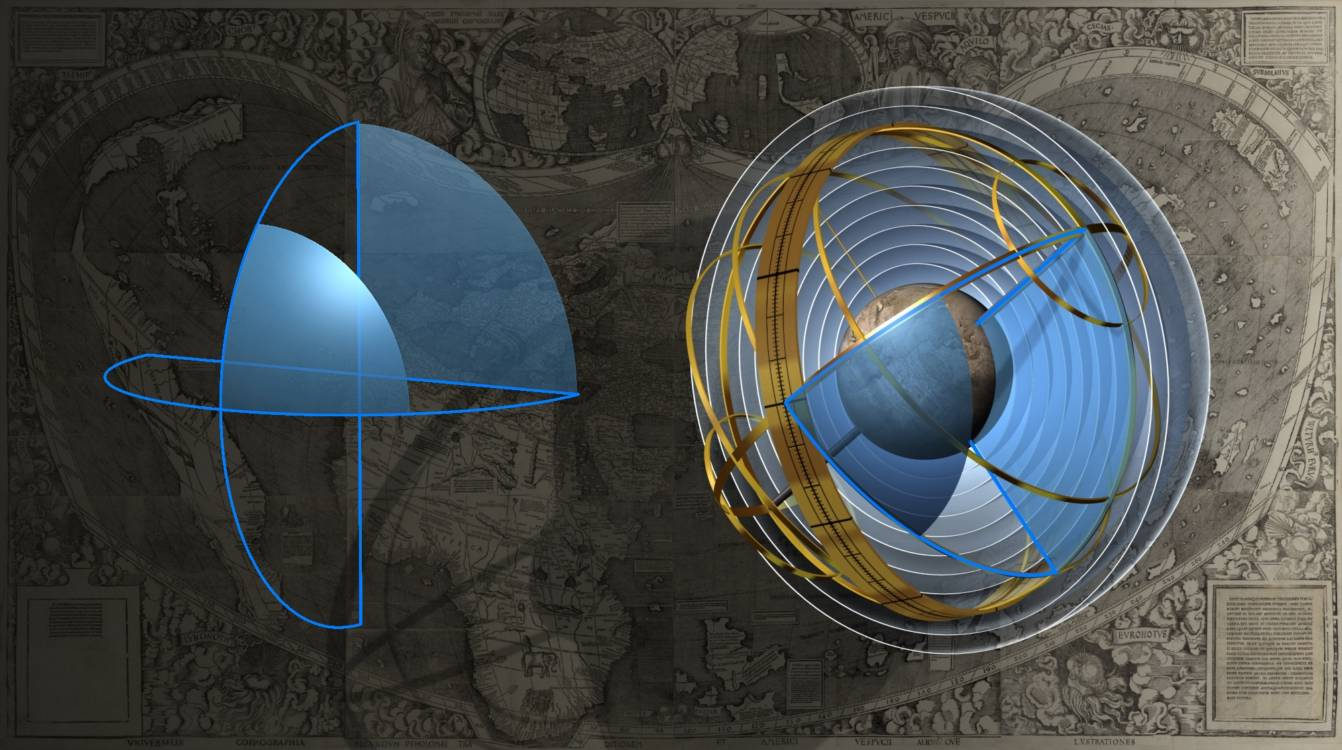“A right angle is an angle formed by one line falling upon another in such a way that the two angles made on either side are the same. If a right angle is formed by straight lines it is called plane. If the angle is formed by curved lines it is called spherical.” (Cosmographiae introductio, chap. I - Hessler 2008)